# Dynamic Input during Edge Triggering!

#### typloshionk

Joined Aug 31, 2020
12
Hi everyone,
I have a confusion regarding edge-triggered flipflops. In the diagram attached below, I'm confused what to take the value of J and K as input since it is changing at the very edge of the area 3. If we are considering negative-edge triggering, will we take J and K as 0 or as 1? If the same situation happens at the positive edge of the cycle, will it affect the result?

P.S: this is my first time posting here, so apologize if I missed something or posted this in the wrong section.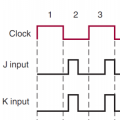#### Deleted member 115935

Joined Dec 31, 1969
0
Can you give us some background please
is this home work, whats the actual question they are asking,
In the real world, clocked JK flip flops are unusual.

#### MrChips

Joined Oct 2, 2009
28,527
As the name suggests, any edge triggered device, rising edge or falling edge trigger, the inputs are interrogated on the edge of the clock. Hence, in your timing diagram shown, J and K inputs will be interrogated as 0.

The outcome will be uncertain at clock pulse 3 with falling edge trigger circuit.

#### MrChips

Joined Oct 2, 2009
28,527
Can you give us some background please
is this home work, whats the actual question they are asking,
In the real world, clocked JK flip flops are unusual.
On the contrary, clocked J-K flip-flops are the norm. There are no J-K flip-flops that use no clock input as far as I am aware.

•ericgibbs

#### typloshionk

Joined Aug 31, 2020
12
Can you give us some background please
is this home work, whats the actual question they are asking,
In the real world, clocked JK flip flops are unusual.
I'm studying electronics in our 6th sem MechEngg. so this fipflop/latches is completely new to me. I don't know if you can call it a homework question since this is just a portion of the question. Actually, they asked to find the output Q of this entire waveform for positive triggering. So I'm confused that when the inputs change(high to low) at the very rising/falling edge then what should we take as the value of input to use in the truth table.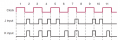#### MrChips

Joined Oct 2, 2009
28,527
Firstly, you need to know the behaviour of J-K flip-flops.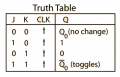Since you are told that the flip-flop in question is positive triggering (rising edge triggered), ignore what happens on the falling edge.

Write down what you observe at the J and K inputs on each rising edge (leading edge) of the clock, from pulse 1 to 11 in sequence.

Edit: Note that the exercise you have been given is a "trick question".

#### typloshionk

Joined Aug 31, 2020
12
Firstly, you need to know the behaviour of J-K flip-flops.

View attachment 216036

Since you are told that the flip-flop in question is positive triggering (rising edge triggered), ignore what happens on the falling edge.

Write down what you observe at the J and K inputs on each rising edge (leading edge) of the clock, from pulse 1 to 11 in sequence.
I didn't want to ask the homework question directly so changed the positive triggering to negative.

#### MrChips

Joined Oct 2, 2009
28,527
I didn't want to ask the homework question directly so changed the positive triggering to negative.
So this is actually a homework question and therefore you altered the original question.
This makes a huge difference in the answer, both in the behaviour of the flip-flop and how we respond to the question according to AAC forum rules.

#### typloshionk

Joined Aug 31, 2020
12
So this is actually a homework question and therefore you altered the original question.
This makes a huge difference in the output of the answer.
Riiight, sorry for that. Let's suppose the following diagram. For negative triggering what will be the value of J? Will it be 1 since it was 1 as we started the edge of clock or 0 since it is 0 at the end of edge? I'll try to figure out for positive triggering by myself, if not then I can always ask here.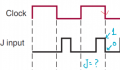#### MrChips

Joined Oct 2, 2009
28,527
The J-input is changing. The answer is J can be any value between the binary values of 0 and 1. In other words, the value is what you get were you to flip a coin.

#### typloshionk

Joined Aug 31, 2020
12
The J-input is changing. The answer is J can be any value between the binary values of 0 and 1. In other words, the value is what you get were you to flip a coin.
Yes, this is what I can't understand; which value to take. For e.g. in the following diagram, what would be the value of Q output at the edge shown since both input J and K are changing.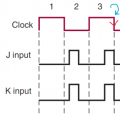#### BobTPH

Joined Jun 5, 2013
6,527
The answer is that it is invalid input and we cannot say what the output is.

In the case of a real device there will be parameters In the datasheet telling you how long the inputs must be stable around the clock pulse. Anything outside those parameters is considered invalid and there is no guarantee of the outputs.

Bob

•typloshionk

#### MrChips

Joined Oct 2, 2009
28,527
There is no in-between answer since the outcome is a binary value.

•typloshionk

#### typloshionk

Joined Aug 31, 2020
12
Right. Thank you everyone for answering, especially MrChips. I think I know what to do now.

#### typloshionk

Joined Aug 31, 2020
12
Edit: Note that the exercise you have been given is a "trick question".
MrChips, if you don't mind can you please tell me what is tricky about this question which you mentioned earlier? It will really help me a lot. Thank you!

#### Papabravo

Joined Feb 24, 2006
20,154
A real JK-flip-flop will have two parameters specified in the datasheet. These two parameters are called "setup time" and "hold time". The setup time is the amount of time before the clock edge that the input is required to be stable and the hold time is the amount of time after the clock edge. These times give you a usable metric to determine the values to use. Setup and hold time violations can lead to meta-stable behavior which as you can surmise is a bad-bad thing.

Last edited:

#### MrChips

Joined Oct 2, 2009
28,527
MrChips, if you don't mind can you please tell me what is tricky about this question which you mentioned earlier? It will really help me a lot. Thank you!
I said it was a "trick question" for two reasons before you told us that you had modified the question.

1) Assuming that the question was about clocking on the rising transition of the clock I saw that there were three occasions when the J and K inputs were in transition. I ignored what was happening on the falling edge of the clock.

2) Since only rising transitions of the clock were to be considered you only needed to look at the odd cycles, 1, 3, 5, ... 11.

A question of this nature is usually interested in the operation of J-K flip-flops under valid levels of J and K inputs. Hence I posted the truth table on J-K flip-flops.

In turns out that that is not the goal of the question. The goal of the question is to find out your knowledge of J-K flip-flops when the J and K inputs are in transistion. We have answered that question.

#### typloshionk

Joined Aug 31, 2020
12
Thank you MrChips for the detailed answer. Now, I have understood that these transitions at the input (for both positive and negative) edge triggering will give us an indeterminate output.
I was led to confusion by the answers/articles posted on the internet. For e.g. on Chegg, someone posted the answer for exact same question but in that, he considered the value of input as the value which is at the start of clock edge during transition stages. Similarly, on another article, someone used the exact opposite approach (i.e. considering the input value at the end of clock edge) although for different waveforms of inputs but exact same setting (transitions, jk flipflop etc).

#### WBahn

Joined Mar 31, 2012
28,495
In most well-designed systems, the J and K signals are derived from the outputs of other flip flops and all of the flip flops are clocked with the same clock. The fastest that a signal can get from one flip flop's output to the another flip flop's input is the propagation delay of the flip flop (it would have to be directly connected to it, which is not unusual). Well-designed flip flops have hold times that are shorter than their propagation delays specifically for this reason. As a result, in timing diagrams all of the signals are often shown to be changing at the clock edges and it is understood that input signals are stable at the value before the clock edge until sufficiently after the clock edge to satisfy hold time requirements.

However, the fact that the problem you are given has the J and K input changing away from the clock edges calls this assumption into question since they are clearly asynchronous. Therefore you can approach it a couple of ways. First, you can say that the input state is unknown and see if it makes a difference. If it doesn't, then life is good. If it does make a difference, then the output is unknown after the transition. Another approach would be to state your assumption regarding the find details of the signal changes near the clock edges.

•typloshionk